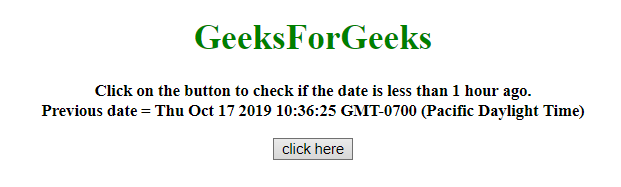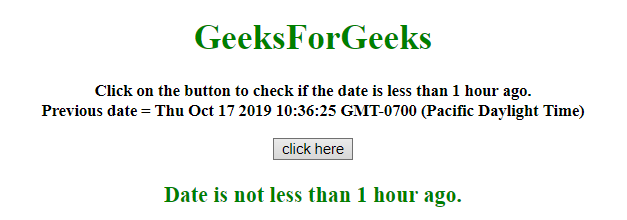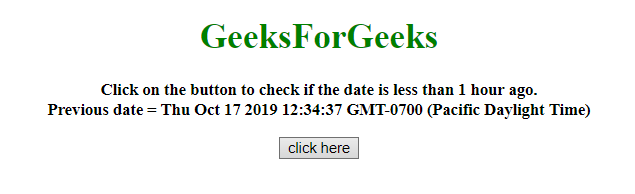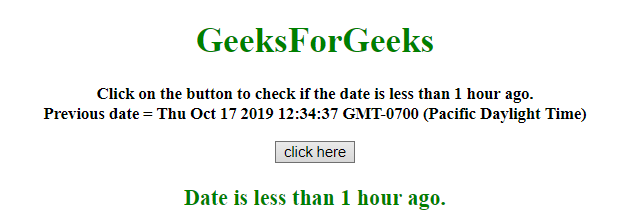# How to check if date is less than 1 hour ago using JavaScript ?

Given a date and the task is to check whether the given date is less than 1 hour ago or not with the help of JavaScript.

Approach 1:

• Count the milliseconds of the difference between the current and prev_date.
• If those are greater than milliseconds in 1 hour, then it returns false otherwise returns true.

Example: This example implements the above approach.

 `  ` `<``html``>  ` ` `  `<``head``>  ` `    ``<``title``>  ` `        ``How to check if date is less than ` `        ``1 hour ago using JavaScript ? ` `    `` ` `  ` ` `  `<``body` `style` `= ``"text-align:center;"``>  ` `     `  `    ``<``h1` `style` `= ``"color:green;"` `>  ` `        ``GeeksForGeeks  ` `    `` ` `     `  `    ``<``p` `id` `= ``"GFG_UP"` `style` `=  ` `        ``"font-size: 15px; font-weight: bold;"``> ` `    `` ` `     `  `    ``<``button` `onclick` `= ``"gfg_Run()"``>  ` `        ``click here ` `    `` ` `     `  `    ``<``p` `id` `= ``"GFG_DOWN"` `style = "color:green; ` `        ``font-size: 20px; font-weight: bold;"> ` `    `` ` `     `  `    ``<``script``> ` `        ``var el_up = document.getElementById("GFG_UP"); ` `        ``var el_down = document.getElementById("GFG_DOWN"); ` `        ``var prev_date = new Date(); ` `        ``var d = new Date(); ` `     `  `        ``Date.prototype.addHours = function(h) {  ` `            ``this.setTime(this.getTime() + (h*60*60*1000));  ` `            ``return this;  ` `        ``} ` `         `  `        ``prev_date.addHours(-2); ` `        ``el_up.innerHTML = "Click on the button to " ` `                ``+ "check if the date is less than " ` `                ``+ "1 hour ago.<``br``>Previous date = " ` `                ``+ prev_date; ` `         `  `        ``function gfg_Run() { ` `             `  `            ``// Hour in milliseconds ` `            ``var ONE_HOUR = 60 * 60 * 1000; ` `             `  `            ``if ((d - prev_date) < ``ONE_HOUR``) { ` `                ``el_down.innerHTML` `= ``"Date is less"` `                            ``+ " than 1 hour ago."; ` `            ``} ` `            ``else { ` `                ``el_down.innerHTML` `= ``"Date is not "` `                        ``+ "less than 1 hour ago."; ` `            ``} ` `        ``}          ` `    ``  ` `  ` ` `  ` `

Output:

• Before clicking on the button:• After clicking on the button:Approach 2:

• Subtract 1 hour milliseconds from current time.
• Compare the milliseconds of the current and prev_date.
• If those are greater than milliseconds in 1 hour, then it return false otherwise returns true.

Example 2: This example uses the approach as discussed above.

 `  ` `<``html``>  ` ` `  `<``head``>  ` `    ``<``title``>  ` `        ``How to check if date is less than ` `        ``1 hour ago using JavaScript ? ` `    `` ` `  ` ` `  `<``body` `style` `= ``"text-align:center;"``>  ` `     `  `    ``<``h1` `style` `= ``"color:green;"` `>  ` `        ``GeeksForGeeks  ` `    `` ` `     `  `    ``<``p` `id` `= ``"GFG_UP"` `style` `=  ` `        ``"font-size: 15px; font-weight: bold;"``> ` `    `` ` `         `  `    ``<``button` `onclick` `= ``"gfg_Run()"``>  ` `        ``click here ` `    `` ` `     `  `    ``<``p` `id` `= ``"GFG_DOWN"` `style = "color:green; ` `        ``font-size: 20px; font-weight: bold;"> ` `    `` ` `     `  `    ``<``script``> ` `        ``var el_up = document.getElementById("GFG_UP"); ` `        ``var el_down = document.getElementById("GFG_DOWN"); ` `        ``var prev_date = new Date(); ` `        ``var d = new Date(); ` `         `  `        ``const check = (date) => { ` `            ``const HOUR = 1000 * 60 * 60; ` `            ``const anHourAgo = Date.now() - HOUR; ` `            ``return date > anHourAgo; ` `        ``} ` `        ``el_up.innerHTML = "Click on the button to check" ` `                    ``+ " if the date is less than 1 hour" ` `                    ``+ " ago.<``br``>Previous date = " + prev_date; ` `         `  `        ``function gfg_Run() { ` `            ``var c = check(prev_date); ` `             `  `            ``if (c) { ` `                ``el_down.innerHTML = "Date is less " ` `                            ``+ "than 1 hour ago."; ` `            ``} ` `            ``else { ` `                ``el_down.innerHTML = "Date is not less" ` `                            ``+ " than 1 hour ago."; ` `            ``} ` `        ``}          ` `    ``  ` `  ` ` `  ` `

Output:

• Before clicking on the button:• After clicking on the button:My Personal Notes arrow_drop_upCheck out this Author's contributed articles.

If you like GeeksforGeeks and would like to contribute, you can also write an article using contribute.geeksforgeeks.org or mail your article to contribute@geeksforgeeks.org. See your article appearing on the GeeksforGeeks main page and help other Geeks.

Please Improve this article if you find anything incorrect by clicking on the "Improve Article" button below.